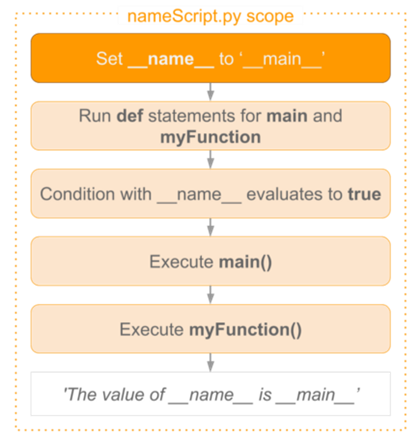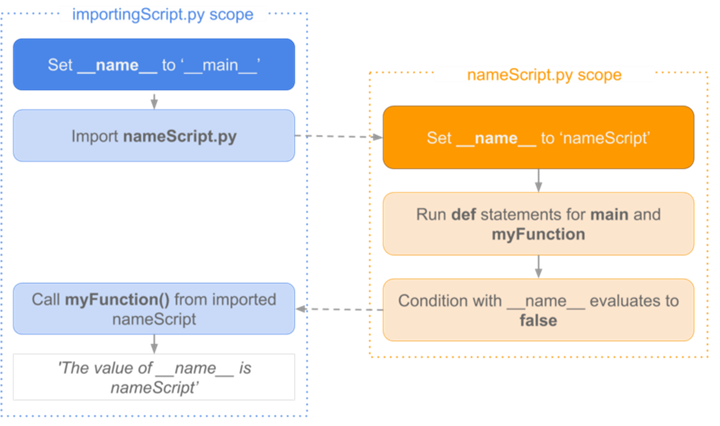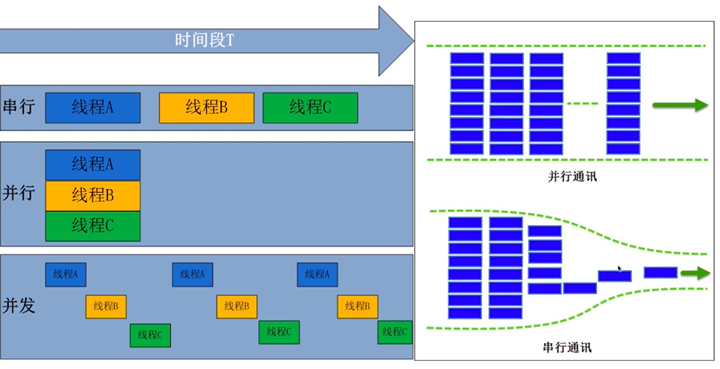Python中的if __name__ == ‘__main__’

if __name__ == "__main__":
print("Hello World!")程序入口

if __name__ == “__main__”: 本质就是一个if判断，但它又不是一个简单的if判断。即当.py文件运行时，__name__ 是’__main__’ 时运行下面if下的代码，则否不运行。当然你也可以把代码写成这样：

if __name__ == "__main__":
print("Hello World!")
else:
print("Hello Mars!")

__name__是什么？

__name__是Python内置的变量，它是每个 Python 模块必备的属性，但它的值取决于你是如何执行这段代码的。

• 当你直接执行一段脚本的时候，这段脚本的 __name__变量等于 ‘__main__’
• 当这段脚本被导入其他程序的时候，__name__ 变量等于脚本本身的名字

def myFunction():
print('The value of __name__ is ' + __name__)

def main():
myFunction()

if __name__ == '__main__':
main()importingScript.py 的内容如下：

import nameScript as ns

ns.myFunction()__name__可以显示包路径

a
├── b
│   ├── c.py
│   └── __init__.py
└── __init__.py
d.py

c.py文件中的代码：

print(__name__)

d.py文件中的代码：

from a.b import c

a.b.c

代码目的：测试模块里函数

def safe_division(a, b):
if b == 0:
return None

return a/b

if __name__ == '__main__':
print(safe_division(10, 5) == 2)
print(safe_division(10, 0) == None)如何理解Python装饰器Python协程与异步